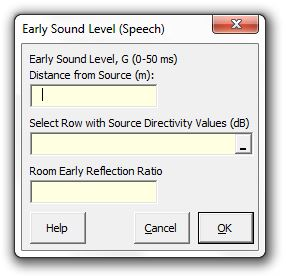### Strutt Help

Early Sound Level (Speech)    1/1, 1/3

Strutt|Auditorium Acoustics|Early Sound Level (Speech) calculates the early sound level (G_(0-50ms)) in a drama auditorium using Barron's modified revised theory.The modified version of revised theory allows for the case of a proscenium theatre in which a proportion of both the early and late sound energy will escape into the flytower. The source directivity (Q) becomes significant when considering natural speech in a theatre.

In addition, the 'early reflection ratio' (N) is used to describe the 'reflection gain' provided by the surfaces of the theatre. The early reflection ratio is the effective strength of all early energy (up to 50 ms), including the direct sound relative to the direct sound.

Hence N=1 indicates that only the direct sound is present. N=2 indicates that the early reflections are equal to the direct sound. N=3 indicates that the cumulative early reflections have twice the strength of the direct sound.

In short, a theatre with a reflection ratio of N will have N-1 strong early reflections.

Refer Section 7.4 and 7.6 Barron for a more-detailed discussion of the early reflection ratio.

The directivity index DI is entered in values of decibels (following Strutt's sign conventions), but is converted to geometric directivity factor Q=10^((DI)/10) for calculation.

Barron provides some indicative values for vocal directivity as a function of angle, as follows:

 Angle of listener to speaker: 0° 45° 90° 135° 180° Directivity index (DI) +3.0 +2.3 -0.6 -3.6 -1.6 Directivity factor (Q) 2.0 1.67 0.87 0.44 0.70

Note that the resulting level is expressed as strength (G) and can be converted to 'relative loudness' (i.e. relative to the source L_W) by subtracting 31 dB.

The early sound is calculated using the following relationship:

e_r = (100 Q N )/r^2, where

r is the distance from the source.
Q is the geometric directivity factor.
N is the early reflection ratio.

Strutt calculates the total early sound level as:

G_(0-50ms)=10log(e_r)

Reference: Barron Auditorium Acoustics and Architectural Design, 2nd edition, Appendix D.3